# TUTORIAL ON SUPERCUBE SOLVING (2) (Solving Odd-Order Supercubes)

In this webpage, I will show how to solve odd-order Supercubes. The solution procedure will be divided into 7 steps with some steps subdivided into sub-steps. If you are trying to solve a 3rd-order Supercube, then you may jump straight to Step VI. Otherwise, please start from Step I below.

## Step I: Match the Center Pieces on the first 4 Faces

In the first step, you try to match the center pieces on any 4 faces, i.e. move the non-core center pieces to the correct positions relative to the core center pieces. Although you can freely choose any 4 faces in this step, to facilitate the following discussion, I assume that these 4 faces are the white, orange, blue and green faces. The method for accomplishing this step is basically the same as that for matching the center pieces on the first 4 faces of a Rubik's Cube, and I assume you already know how to do it. If you don't, please watch this video before you continue reading. Please note that there is not a fixed algorithm for this step and so you must figure this part out for yourself, before you can proceed to the next step.

Of course, there is a fundamental difference between a Rubik's Cube and a Supercube: for a Rubik's Cube, there is no difference among the four center pieces belonging to the same orbit and in the same color. But for a Supercube, these center pieces differ from one another and cannot be placed at the wrong positions. I will now illustrate this point using the following figure of a 5th-order Supercube: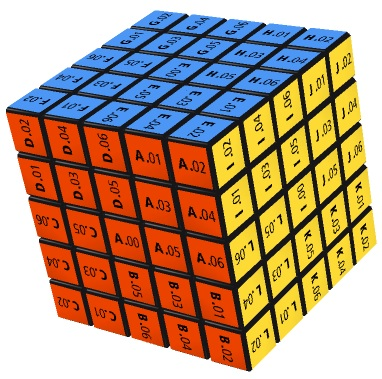In the figure above, the red "03" orbit contains 4 center pieces labelled "A.03", "B.03", "C.03" and "D.03". Without these labels, we will have a Rubik's Cube and we can swop the positions of these 4 center pieces among themselves. But when solving the Supercube, you cannot mess up the positions of these center pieces. This is why we say that solving a Supercube is harder than solving a Rubik's Cube. But despite this complication, this step of solving the Supercube does not require new skills or new formulas other than those required for solving the Rubik's Cube.

## Step II: Match the Center Pieces on the 5th Face

Upon completion of Step I, the Supercube should look like this: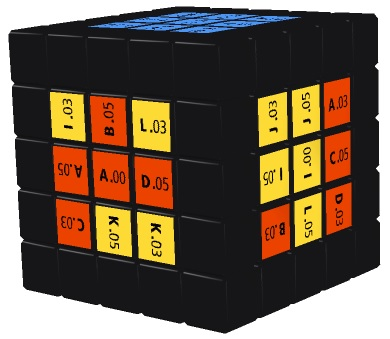In the figure above, only the center pieces on the red and yellow faces remain unmatched. Since all the center pieces on the other 4 faces are matched, all red and yellow center pieces are now located on these two remaining faces. In this step, we select one face from these two remaining faces and try to match the center pieces on this face. To be specific, suppose we select the yellow face. We then try to move the yellow center pieces located on the red face to the appropriate positions on the yellow face one by one, by using the following "(XYZ) Formulas":

 Clockwise (XYZ) Formula: Ux−1 R Uz−1 R−1 Ux R Uz R−1 (1) Anticlockwise (XYZ) Formula: Ux−1 R−1 Uz−1 R Ux R−1 Uz R (2)

The conditions and examples of use of these two formulas are given in TUTORIAL ON SUPERCUBE SOLVING (1): Basic Notions. Note that when doing this step, if you find that some yellow center pieces are located at wrong positions on the yellow face, you may need to move these yellow pieces to the red face first, and then move these yellow pieces back to the correct positions on the yellow face.

## Step III: Match the Center Pieces on the 6th Face

Upon completion of Step II, the Supercube should look like this (which is now illustrated by a 7th-order Supercube):In the figure above, although all red center pieces are now located on the red face, not all of them are at the correct positions. In this step, we will use the (XYZ) Formulas to move the red pieces from their incorrect positions to correct posistions. But before doing this, we must check whether each orbit of the non-core center pieces on the red face has an even relative parity (to be defined below). This is because the (XYZ) Formulas can only take the desirable effect on orbits with an even relative parity. Thus, to accomplish this step, you need to go through the following three sub-steps.

### Sub-step 1: Check whether all Orbits of Non-core Center Pieces have Even Relative Parities

For Supercubes, we can define two notions of parity: absolute parity and relative parity. Since the definitions are quite technical, I will not go into the details. But fortunately, for the purpose of Step III, there is an easy way to define the relative parity of each orbit of non-core center pieces on the red face. You first list the 4 alphabets ("A", "B", "C" and "D") that appear in each orbit in clockwise direction, with the alphabet that appears on the top right hand side of the string ".00" in the core center piece being listed first. Note that here "top right hand side" is relative to the string ".00". Under different orientations of the core center piece, "top right hand side" may mean differently. After listing the alphabets, you then determine how many transpositions you need to make in order to transform this list to "ABCD". By "transposition" I mean interchanging the positions of any two alphabets. If the number of transpositions is an even number, the relative parity is even, otherwise it is odd. If all orbits have even parities, you can then jump to Sub-step 3. Otherwise, you proceed to Sub-step 2.

For illustration, I use the "05" point piece orbit of the 7th-order Supercube shown above as an example. Since the core center piece "A.00" in the figure has been rotated clockwise by 90°, the "05" point piece that appears on the top right hand side of the string ".00" is the "A.05" piece (rather than the "C.05" piece). Starting from the "A.05" piece, the alphabet list of the "05" orbit is "ADBC". Since you need two transpositions to transform this to "ABCD" (by interchanging "D" and "B" first and then interchanging "D" and "C"), the relative parity of the "05" orbit is even. The following table lists the alphabet lists of the six orbits of non-core center pieces on the red face and their relative parities. You should check whether you understand how to determine the relative parities:

OrbitTypeAlphabet ListRelative Parity
05
point piece
even
07
point piece
CDAB
even
11
cross piece
odd
10
cross piece
odd
08
paired piece
(matched up with the "04" orbit)
CDAB
even
04
paired piece
(matched up with the "08" orbit)
even

The table shows that the "10" and "11" orbits both have odd relative parities. Therefore, you need to go through Sub-step 2.

Before introducing the next sub-step, I have to point out the following important facts which warrnat special attention:

Fact 1: The relative parity of each point piece orbit is always even.

Fact 2: The orbits of paired pieces that are matched up with each other have the same relative parity.

Thanks to these two facts, the checking process in this sub-step can now be greatly simplified. First, you do not need to check the relative parities of the point piece orbits. Second, for each pair of paired piece orbits that are matched up with each other, you only need to check the relative parity of one orbit from among the pair. For example, for the 7th-order Supercube shown above, you only need to check the relative parities of the "11", "10" and "08" (or "04") orbits.

### Sub-step 2: Ensure all Orbits of Non-core Center Pieces have Even Relative Parities

In this sub-step, you only need to focus on the cross pieces in the right half of the red face. For convenience, let's call these pieces the focused pieces. For example, in the 7th-order Supercube shown above, the focused pieces are the "B.11" and "D.10" pieces encircled by the yellow frame in the following figure: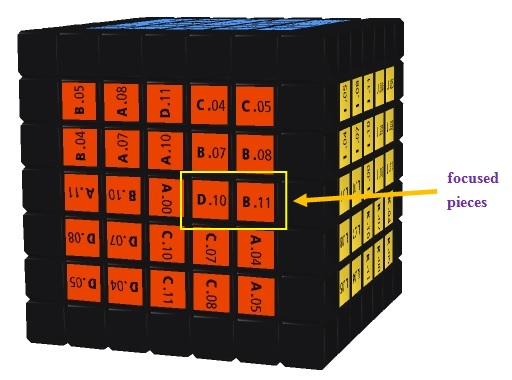To accomplish the objective of this sub-step, you first need to rotate the whole Supercube so that the red face faces upward, and then apply the following One Side Swapping Formula to every non-core inner layer in the right half of the red face that contains a focused piece orbit with odd relative parity:

One Side Swapping Formula: Lx−1 U2 Lx−1 U2 F2 Lx−1 F2 Rx U2 Rx−1 U2 Lx2     (3)

The subscripted variable x represents the non-core inner layer that you need to apply this formula to. Note that by just focusing on the focused piece orbits as described above, you will automatically take care of all non-core center piece orbits, i.e. you will ensure that all non-core center piece orbits have even relative parities.

Using the 7th-order Supercube shown above as an example, you may recall (by referring to the table above) that the "11" and "10" orbits both have odd relative parities. This means that the first and second inner layers in the right half of the red face each contains a focused piece orbit with odd relative parity. So you need to apply formula (3) to these two inner layers, i.e. apply (3) twice with the variable x substituted by 1 and 2, successively; or apply (3) once by moving these two inner layers together. After the above operation, the Supercube will look like this:You may now verify that all orbits of non-core center pieces in the Supercube above have even relative parities.

### Sub-step 3: Use the (XYZ) Formulas to restore the Non-core Center Piece Orbits to Normal

In this sub-step, "restore to normal" means restoring the alphabet list to "ABCD". When using the (XYZ) Formulas, you have to make use of a center piece on the other face by moving it temporarily to the red face and finally move it back to its original position. For readers' convenience, I list the restoration procedure of each possible situation in the table below (if the 6th face that you choose is not the red face, then you have to change the alphabets in the following table to the corresponding alphabets. For example, if you choose the yellow face as the 6th face, then you have to change A, B, C, D to I, J, K, L respectively):

Table 1
Alphabet ListRestoration Procedure
ABCD
no need to restore
ABDC
odd relative parity
ACBD
odd relative parity
ACDB
(B#D) → (#BC)
(D#B) → (#DC)
odd relative parity
BACD
odd relative parity
(A#B) → (DAC) → (#DA)
(A#C) → (#AB)
BCDA
odd relative parity
BDAC
odd relative parity
BDCA
(D#B) → (#DA)
CABD
(C#A) → (#CB)
odd relative parity
odd relative parity
CBDA
(C#A) → (#CD)
CDAB
(A#B) → (#AC) → (D#B) → (#DC)
CDBA
odd relative parity
DABC
odd relative parity
DACB
(B#D) → (#BA)
DBAC
(C#A) → (#CD)
DBCA
odd relative parity
DCAB
odd relative parity
DCBA
(A#D) → (CA#) → (#CB)

The left column of the table above lists all the 24 possible permutations of A, B, C and D. Among these permutations, one permutation does not need restoration, while 12 permutations have odd relative parities and must be transformed to even relative parities using the method introduced in Sub-step 2 first. The remaining 11 permutations can be restored to normal using the (XYZ) Formulas. The right column of the table above tells you the restoration procedure, where "#" represents any suitable center piece on another face of the Supercube. Note that when using the table above, sometimes you need to rotate the red face or another face to ensure that the center pieces in question satisfy the use condition for the (XYZ) Formulas. I now use the following figure to illustrate how to use the table above:The figure above shows that the alphabet list of the "05" orbit is "BCAD". According to Table 1, you have to do (A#C) first, i.e. you have to move the "A.05" piece to the position of a suitable piece on another face (which is denoted "#"), move "#" to the position of the "C.05" piece, and move the "C.05" piece to the position of the "A.05" piece. Now suppose you choose the "I.05" piece on the yellow face as "#", i.e. by substituting "I" into "#". After using the (XYZ) Formulas appropriately, you should obtain the following result (verify that the following figure does give the desired result (AIC)):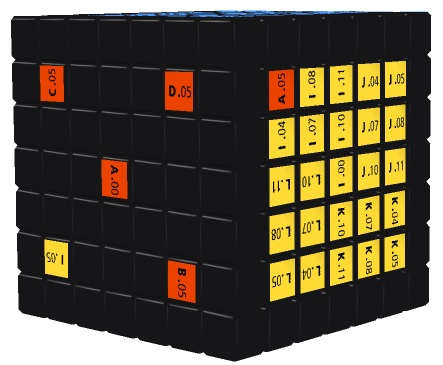According to Table 1, you next have to do (#AB), i.e. (IAB). Since in the figure above, the "I,05" and "A.05" pieces are not at corresponding positions and the "I.05" and "B.05" pieces lie on the same horizontal line, to satisfy the use condition for the (XYZ) Formulas, you have to rotate the red face by 90° clockwise, and then use the (XYZ) Formulas appropriately. After this, you should obtain the following result: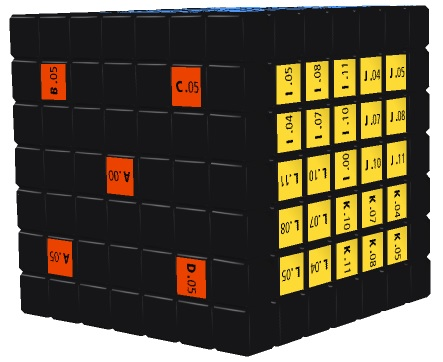In the figure above, the alphabet list of the "05" orbit has been restored to "ABCD" and the "I.05" piece has also been returned to the yellow face. By following the above procedures, you will be able to restore all orbits of non-core center pieces on the red face to normal and finally complete this sub-step as well as Step III.

## Step IV: Match the Edge Pieces on the first 10 Edges

In this step, you try to match the edge pieces on any 10 edges of your choice, i.e. move the non-core edge pieces to the correct positions relative to the core edge pieces. Just like Step I, the method for accomplishing this step is basically the same as that for matching the edge pieces on the first 10 edges of a Rubik's Cube, and I assume you already know how to do it. If you don't, please watch this video before you continue reading. Note that there is not a fixed algorithm for this step and so you must figure this part out for yourself, before you can proceed to the next step.

## Step V: Match the Edge Pieces on the remaining 2 Edges

If you are lucky enough, you will be able to match the edge pieces on all the 12 edges by using the method in Step IV. But very often you are not so lucky, and you have to use some formulas to swap / flip some edge pieces. These include the One Side Swapping Formula given above, i.e. formula (3) and the following four formulas:

Two Side Swapping Formula: One Side Flipping Formula: F2 Lx−1 F B−1 R2 F−1 B Rx Lx F B−1 R2 F−1 B Rx−1 F2 (4) Rx2 B2 U2 Lx U2 Rx−1 U2 Rx U2 F2 Rx F2 Lx−1 B2 Rx2 (5) U−1 F R−1 U F−1 Rx−1 Lx F U−1 R F−1 U Rx Lx−1 (6) F U−1 R Fm R−1 U F−1 R U−1 Fm−1 U R−1 (7)

The effects of these formulas are given in TUTORIAL ON SUPERCUBE SOLVING (1): Basic Notions. Before using these formulas, you have to make sure that the remaining unmatched 2 edges are the front-top edge and back-top edge of the Supercube. You can always do this by appropriately rotating the outer surfaces of the cube. I use the following figure to illustrate how to use the formulas above: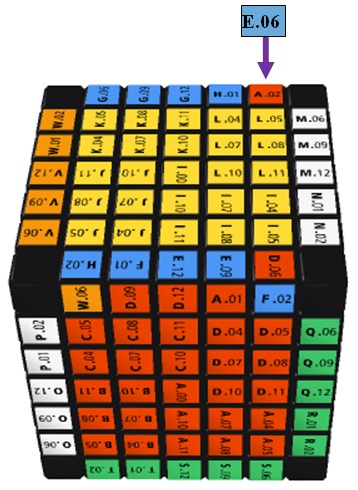To match the edge pieces on the front-top and back-top edges of the Supercube shown above, you may first substitute 1 into the variable x and use the One Side Flipping Formula (5). This will flip and swap the "W.06-H.02" and "F.02-D.06" edge pieces. You then substitute 1 into the variable x and use the One Side Swapping Formula (3). This will swap the "E.06-A.02" and "W.06-H.02" edge pieces and thus yield the desired result.

Some readers may worry that by using formulas (3) - (7), you may screw up the centers that have been matched in the previous steps. But please rest assured that this will not be the case. As a matter of fact, formulas (4), (6) and (7) will not screw up any center (try and see). Although formulas (3) and (5) will screw up the top center, if you use them one after the other on the same non-core inner layer, the net effect is that the top center will not get screwed up (try and see). And when solving an odd-order Supercube, it will always be the case that formulas (3) and (5) are used one after the other on the same non-core inner layer.

## Step VI: Solve the Corners and Edges

Upon completion of Step V, the Supercube should look like this:If you view the edge pieces on each edge as a whole and the center pieces in each center as a whole, then the Supercube above is no different from a 3rd-order Supercube. Therefore, you can use the same method of solving a 3rd-order Rubik's Cube to solve the corners and edges of the Supercube. I assume that you already know how to do this. If you don't, please consult 7 Steps Solution Guide. Note that when doing this step, you do not have to worry about the orientation of the centers, because this will be taken care of in the next step. Just forget the symbols on the center pieces for the time being.

## Step VII: Orient the Centers correctly

Upon completion of Step VI, the Supercube should look like this (which is now illustrated by a 5th-order Supercube):While the Supercube above seems to be solved, careful examination of the center pieces will show that not all the 6 centers are oriented correctly (although all non-core center pieces are matched correctly with the core center pieces). For example, the red centers and yellow centers both deviate from the correct orientation by 90° clockwise. To orient the centers correctly, you can rotate the centers by using the following formulas:

 Two Center Rotation Formula: L−1 R F−1 B U−1 D Ln D−1 U B−1 F R−1 L U−n (8) One Center Rotation Formula: (U R L U2 R−1 L−1)2 (9)

The effects of these formulas are given in TUTORIAL ON SUPERCUBE SOLVING (1): Basic Notions. Note that by using the formulas shown above appropriately, you will be able to handle all possible situations.

For instance, to orient the red and yellow faces in the figure above correctly, you can do the following. Rotate the whole Supercube so that the red face faces left and the yellow face faces upward. Then substitute 1 into the variable n and use the Two Center Rotation Formula (8). After that, the Supercube should look like this: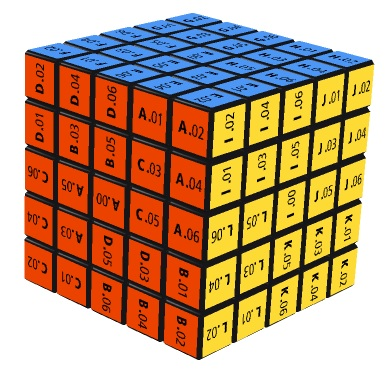The figure above shows that the yellow center is now oriented correctly while the red center deviates from the correct orientation by 180°. You should then rotate the whole Supercube so that the red face faces upward. Then use the One Center Rotation Formula (9). After that, the red center will also be oriented correctly.

Go to Supercube Links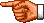Go toMy Personal Homepage (English Version)
Go toMy Personal Homepage (Chinese Version)